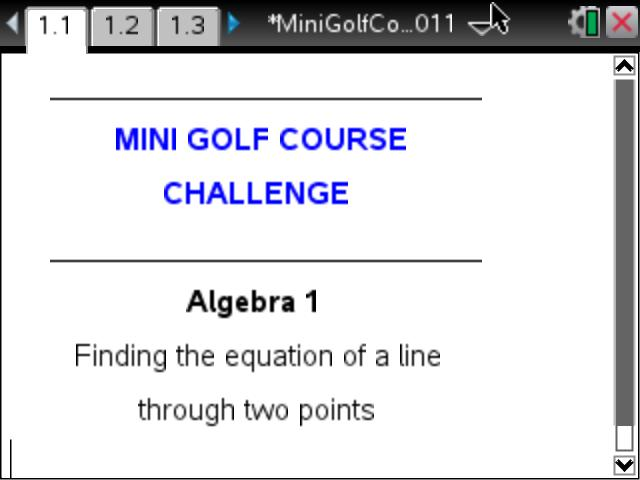# Activities

•• ##### Subject Area

• Math: Algebra I: Linear Functions

• ##### Author6-8
9-12

45 Minutes

• ##### Device
•TI-Nspire™ CX/CX II
• TI-Nspire™ Navigator™
• TI-Nspire™

3.2

## Linear Equation Games Unit: Activity #5 Mini Golf Course Challenge#### Activity Overview

This activity involves students using the coordinates of two points to find the equation that runs from the 'tee' to the golf 'hole'.

This is the fifth activity in the Linear Games Unit. This is the next step where students are to create a linear equation that runs through the two points. Some of the 'holes' have 'bumper points' which requires them to find more than one equation.

Note: There is another file by Jens Larson that has the students do the same thing but he uses images of golf holes. This is a good place in the unit to put this file which can be found here.